# ML Aggarwal Class 8 Solutions for ICSE Maths Chapter 7 Percentage Ex 7.3

## ML Aggarwal Class 8 Solutions for ICSE Maths Chapter 7 Percentage Ex 7.3

Question 1.
Find the discount and the selling price, when:
(i) the marked price = ₹575, discount = 12%
(ii) the printed price = ₹12750, discount = $$8 \frac{1}{3}$$%
Solution: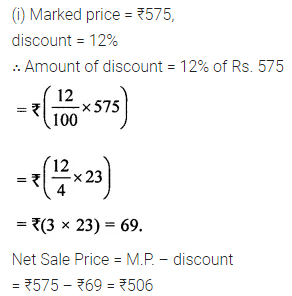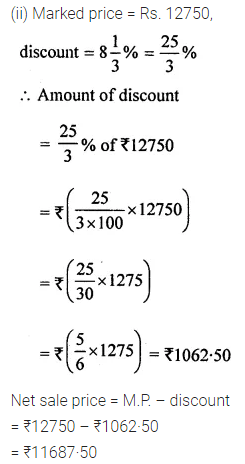Question 2.
Find the discount and the discount percentage, when :
(i) marked price = ₹780, selling price = ₹721·50
(ii) advertised price = ₹28500, selling price = ₹24510
Solution:Question 3.
A notebook is marked at ₹30. Find the price a student pays for a dozen notebooks if he gets 15% discount.
Solution: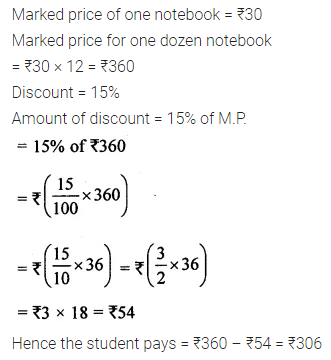Question 4.
A dealer gave 9% discount on an electric fan and charges ₹ 728 from the customer. Find the marked price of the fan.
Solution:Question 5.
The list price of an article is ₹800 and a dealer is selling it at a discount of 20%. Find :
(i) the selling price of the article.
(ii) the cost price of the article if he makes 25% profit on selling it.
Solution:Question 6.
A shopkeeper marks his goods at such a price that would give him a profit of 10% after allowing a discount of 12%. If an article is marked at ₹2250, find its :
(i) selling price
(ii) cost price.
Solution: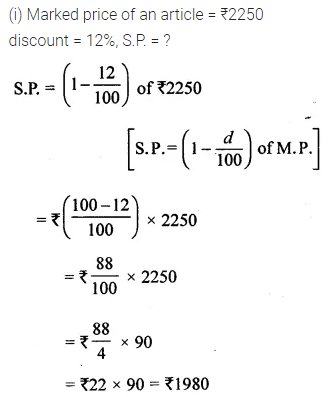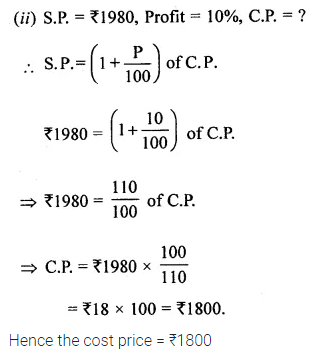Question 7.
A shopkeeper purchased a calculator for ₹650. He sells it at a discount of 20% and still makes a profit of 20%. Find :
(i) the selling price
(ii) marked price
Solution: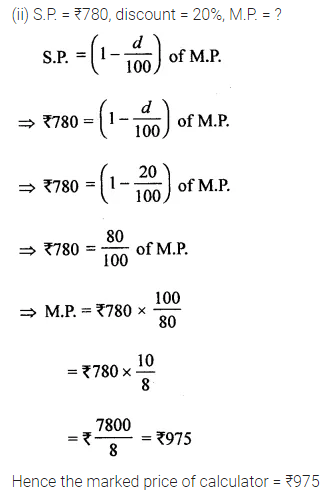Question 8.
A shopkeeper buys a dinner set for ₹ 1200 and marks it 80% above the cost price. If he gives 15% discount on it, find :
(i) the marked price
(ii) the selling price
(iii) his profit percentage.
Solution:Question 9.
The cost price of an article is ₹1600, which is 20% below the marked price. If the article is sold at a discount of 16%, find :
(i) the marked price
(ii) the selling price
(iii) profit percentage.
Solution: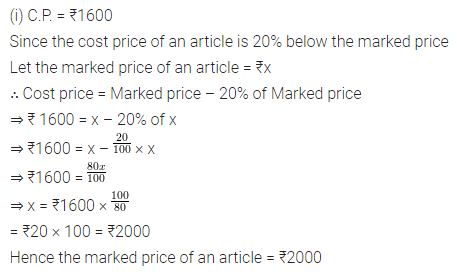Question 10.
A shopkeeper allows 20% discount on his goods and still earns a profit of 20%. If an article is sold for ₹360, find:
(i) the marked price
(ii) tine cost price.
Solution: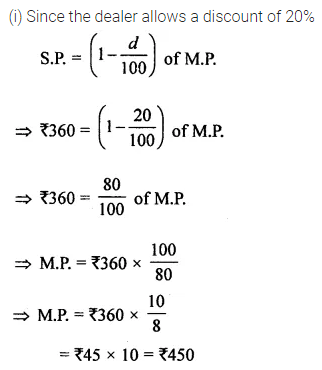Question 11.
The printed price of a refrigerator is ₹28600. A dealer allows two successive discount of 10% and 5%. Find the price which a customer has to pay for the refrigerator.
Solution: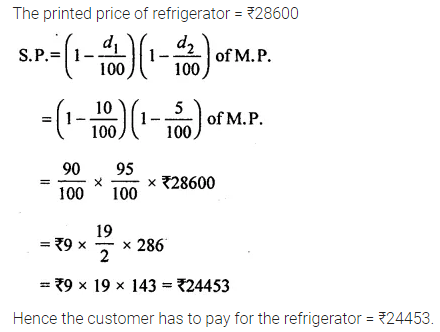Question 12.
Two dealers have marked an article at the same price. The first dealer allows two successive discounts of 15% and 5%. The other allows for a discount of 20% which is the better offer?
Solution: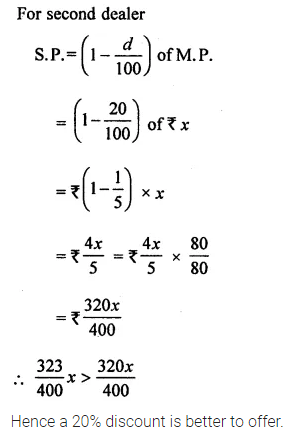Question 13.
Find a single discount equivalent to two successive discounts of 30% and 10%.
Solution: